Courses

# Transition And Diffusion Electrical Engineering (EE) Notes | EduRev

## Electronic Devices

Created by: Cstoppers Instructors

## Electrical Engineering (EE) : Transition And Diffusion Electrical Engineering (EE) Notes | EduRev

The document Transition And Diffusion Electrical Engineering (EE) Notes | EduRev is a part of the Electrical Engineering (EE) Course Electronic Devices.
All you need of Electrical Engineering (EE) at this link: Electrical Engineering (EE)

TRANSITION AND DIFFUSION CAPACITANCE

Electronic devices are inherently sensitive to very high frequencies. Most shunt capacitive effects can be ignored at lower frequencies because the reactance XC=1/2πfC is very large (open-circuit equivalent). This, however, cannot be ignored at very high frequencies. XC will become sufficiently small due to the high value of frequency which introduces a low-reactance “shorting” path. In the PN semiconductor diode, there are two capacitive effects to be considered. In the reverse-bias region we have the transition- or depletion region capacitance (CT), while in the forward-bias region we have the diffusion (CD) or storage capacitance. Recall that the basic equation for the capacitance of a parallel-plate capacitor is defined by C=€A/d, where € is the permittivity of the dielectric (insulator) between the plates of area A separated by a distance d. In the reverse-bias region there is a depletion region (free of carriers) that behaves essentially like an insulator between the layers of opposite charge. Since the depletion width (d) will increase with increased reverse-bias potential, the resulting transition capacitance will decrease. The fact that the capacitance is dependent on the applied reverse-bias potential has application in a number of electronic systems. Although the effect described above will also be present in the forward-bias region, it is over shadowed by a capacitance effect directly dependent on the rate at which charge is injected into the regions just outside the depletion region. The capacitive effects described above are represented by a capacitor in parallel with the ideal diode, as shown in Fig. 1.38. For low or mid-frequency applications (except in the power area), the capacitor is normally not included in the diode symbol.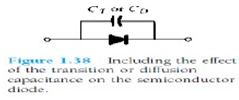Diode capacitances: The diode exhibits two types of capacitances i.e., transition capacitance and diffusion capacitance.

• Transition capacitance: The capacitance which appears between positive ion layer in n-region and negative ion layer in p-region is termed as transition capacitance.
• Diffusion capacitance: This capacitance originates due to diffusion of charge carriers in the opposite regions.

The transition capacitance is very small as compared to the diffusion capacitance.

In the reverse-bias region, the transition capacitance is dominant and is given by: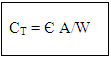where CT  - transition capacitance

A - diode cross sectional area

W - depletion region width

In forward bias, the diffusion capacitance dominates and is given by: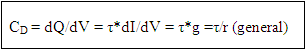where CD  - diffusion capacitance

dQ  - change in charge stored in depletion region

V  - change in applied voltage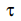- time interval for change in voltage

g  - diode conductance

r  - diode resistance

The diffusion capacitance at low frequencies is given by the formula: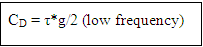The diffusion capacitance at high frequencies is inversely proportional to the frequency and is given by the formula.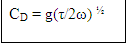Note: The variation of diffusion capacitance with applied voltage is used in the design of varactor.

6 BREAK DOWN MECHANISMS

When an ordinary P-N junction diode is reverse biased, only very small reverse saturation current flows. This current is due to movement of minority carriers. It is almost independent of the voltage applied. However, if the reverse bias is increased, a point is reached when the junction breaks down and the reverse current increases abruptly. This current could be large enough to destroy the junction. If the reverse current is limited by means of a suitable series resistor, the power dissipation at the junction will not be excessive, and the device may be operated continuously in its breakdown region to its normal (reverse saturation) level. It is found that for a suitably designed diode, the breakdown voltage is very stable over a wide range of reverse currents. This quality gives the  diodes many useful applications(in the breakdown region) as a voltage reference source.

The critical value of the voltage, at which the breakdown of a P-N junction diode occurs is called the breakdown voltage. The breakdown voltage depends on the width of the depletion region, which, in turn, depends on the doping level. The junction offers almost zero resistance at the breakdown point.

There are two mechanisms by which breakdown can occur at a reverse biased P-N junction:

1.ava­lanche breakdown.

2.Zener breakdown.

Ava­lanche breakdown
The minority carriers, under reverse biased conditions, flowing through the junction acquire kinetic energy which increases with the increase in reverse voltage. At a sufficiently high reverse voltage (say 5 V or more), the kinetic energy of minority carriers becomes so large that they knock out electrons from the covalent bonds of the semi­conductor material. As a result of collision, the liberated electrons in turn liberate more electrons and the current becomes very large leading to the breakdown of the crystal structure itself. This phenomenon is called the avalanche breakdown. The breakdown region is the knee of the characteristic curve. Now the current is not controlled by the junction voltage but rather by the external circuit.

Zener breakdown

Under a very high reverse voltage, the depletion region expands and the potential barrier increases leading to a very high electric field across the junction. The electric field will break some of the covalent bonds of the semiconductor atoms leading to a large number of free minority carriers, which suddenly increase the reverse current. This is called the Zener effect. The breakdown occurs at a particular and constant value of reverse voltage called the breakdown voltage, it is found that Zener breakdown occurs at electric field intensity of about 3 x 107 V/m.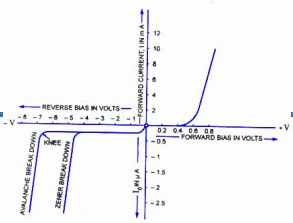Either of the two (Zener breakdown or avalanche breakdown) may occur independently, or both of these may occur simultaneously. Diode junctions that breakdown below 5 V are caused by Zener effect. Junctions that experience breakdown above 5 V are caused by ava­lanche effect. Junctions that breakdown around 5 V are usually caused by combination of two effects. The Zener breakdown occurs in heavily doped junctions (P-type semiconductor mod­erately doped and N-type heavily doped), which produce narrow depletion layers. The ava­lanche breakdown occurs in lightly doped junctions, which produce wide depletion layers. With the increase in junction temperature, Zener breakdown voltage is reduced while the avalanche breakdown voltage increases. The Zener diodes have a negative tempera­ture coefficient while avalanche diodes have a positive temperature coefficient. Diodes that have breakdown voltages around 5 V have zero temperature coefficient. The breakdown phenomenon is reversible and harmless so long as the safe operating temperature is maintained.

Offer running on EduRev: Apply code STAYHOME200 to get INR 200 off on our premium plan EduRev Infinity!

,

,

,

,

,

,

,

,

,

,

,

,

,

,

,

,

,

,

,

,

,

;